# SBI CLERK PRELIMS- QUANTS DAY- 12

Dear Aspirants,

The SBI CLERK Prelims Exam 2018 is one of the important exam and we know you would be searching for the correct planner to devise your strategy. Since the exam pattern is changed , you would have been puzzled about how to study for the exam and make your preparations in the right way. Don’t fret and prepare as you wish but go in the right direction.

The Preliminary Examination of the SBI CLERK 2018 will happen in March/April , so you have to gear up your preparations in the right way to score more in the exams. So attend Daily Quizzes which will enrich your preparations and study and learn new topics which will help you for the exams.

Our Team will be providing the Daily Quizzes on various topics which were based on the previous exams and new pattern questions. This will help you to crack the exam and to achieve your goal. So attend and take the best result which could guide you to find the key of your dreams.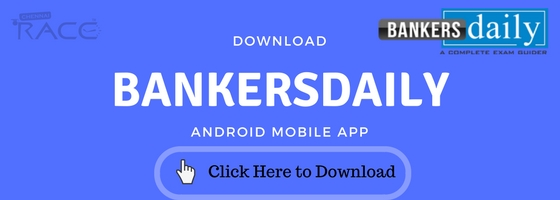SBI Clerk Prelims 2018 Study Planner Day-12

Section : Quantitative Aptitude

Topic : Quadratic Equation

Time: 10 Minutes

D.1–10):Read the Question Carefully and answer the following.
1. x2+3x+2=0

2y2=5y

a) X < Y

b) X > Y

c) X = Y

d) X ≥ Y

e) X ≤ Y

2.(3x)2-7x-20=0

2y2-11y+15=0

a) X < Y

b) X > Y

c) X = Y (or) Cannot be determined

d) X ≥ Y

e) X ≤ Y

3.(10x)2-7x+1=0

35y2-12y+1=0

a) X < Y

b) X > Y

c) X = Y (or) Cannot be determined

d) X ≥ Y

e) X ≤ Y

4.(3x)2 + 7x = 6

6(2y2+1) = 17y

a) X < Y

b) X > Y

c) X = Y (or) Cannot be determined

d) X ≥ Y

e) X ≤ Y

5. x2 – 6x = 7

2y2 + 13y + 15 = 0

a) X < Y

b) X > Y

c) X = Y (or) Cannot be determined

d) X ≥ Y

e) X ≤ Y

6. x2 + 13x + 42 = 0

y2 + 19y + 90 = 0

a) X > Y

b) X ≥ Y

c) X < Y

d) X ≤ Y

e) X = Y (or) Cannot be determined

7. x2– 15x + 60 = 0

y2 – 23y + 132 = 0

a) X > Y

b) X ≥ Y

c) X < Y

d) X ≤ Y

e) X = Y (or) Cannot be determined

8. 82 + 7x + 12 = 0

y2 + 6y + 8 = 0

a) X > Y

b) X ≥ Y

c) X < Y

d) X ≤ Y

e) X = Y (or) Cannot be determined

9. x2 – x – 12 = 0

y2+5y+6 = 0

a) X > Y

b) X ≥ Y

c) X < Y

d) X ≤ Y

e) X = Y (or) Cannot be determined

10. x2 – 8x + 15 = 0

Y2-3y+2 = 0

a) X > Y

b) X ≥ Y

c) X < Y

d) X ≤ Y

e) X = Y (or) Cannot be determined

D.11-20): In each of these questions, two equations (I) and (II) are given. You have to solve both the equations and give answer.

11. 3x2– 29x + 56 = 0

3y2– 5y – 8 = 0

a) If X > Y

b) If X ≥ Y

c) If X < Y

d) If X ≤ Y

e) If X = Y (or) No relation can be established between X and Y.

12. 5x2+ 26x – 24 = 0

5y2– 34y + 24 = 0

a) If X > Y

b) If X ≥ Y

c) If X < Y

d) If X ≤ Y

e) If X = Y (or) No relation can be established between X and Y.

13. x2– 7x = 0

2y2+ 5y + 3 = 0

a) If X > Y

b) If X ≥ Y

c) If X < Y

d) If X ≤ Y

e) If X = Y (or) No relation can be established between X and Y.

14. 7x – 4y = 40

8x + 8y = 8

a) If X > Y

b) If X ≥ Y

c) If X < Y

d) If X ≤ Y

e) If X = Y (or) No relation can be established between X and Y.

15. 15x2– 41x + 14 = 0

2y2– 13y + 20 = 0

a) If X > Y

b) If X ≥ Y

c) If X < Y

d) If X ≤ Y

e) If X = Y (or) No relation can be established between X and Y.

16.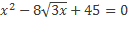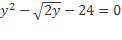a) If X > Y

b) If X ≥ Y

c) If X < Y

d) If X ≤ Y

e) If X = Y (or) No relation can be established between X and Y.

17.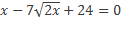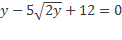a) If X > Y

b) If X ≥ Y

c) If X < Y

d) If X ≤ Y

e) If X = Y (or) No relation can be established between X and Y.

18. 12x2– 17x + 6 = 0

20y2– 31y + 12 = 0

a) If X > Y

b) If X ≥ Y

c) If X < Y

d) If X ≤ Y

e) If X = Y (or) No relation can be established between X and Y.

19. 3x2– 8x + 4 = 0

4y2– 15y + 9 = 0

a) If X > Y

b) If X ≥ Y

c) If X < Y

d) If X ≤ Y

e) If X = Y (or) No relation can be established between X and Y.

20. x2-16x + 63 = 0

y2– 2y – 35 = 0

a) If X > Y

b) If X ≥ Y

c) If X < Y

d) If X ≤ Y

e) If X = Y (or) No relation can be established between X and Y.

Answer key:

1. (a)
2. (c)
3. (d)
4. (e)
5. (b)
6. (a)
7. (c)
8. (e)
9. (e)
10. (a)
11. (b)
12. (d)
13. (a)
14. (a)
15. (c)
16. (e)
17. (b)
18. (d)
19. (e)
20. (a)

You can inspect the other daily quizzes which we post in the SBI CLERK PRELIMS EXAM STUDY PLANNER 2018 from the below given links. Our Team is updating the Daily Quizzes regularly so that, these quizzes will hep your preparations and also to master the SBI CLERK EXAM 2018. Preparing hard is important , by attending our daily quizzes, you are preparing in a smarter way. Also alternately bookmark the below given page , so that this will be useful for your daily preparations.